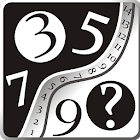Find Next Number

All Android applications categories

All Android games categories# Find Next Number

29 8.2

8.2 Users
rating

## Screenshots

Description

Finding the next number in a sequence of numbers is a typical task in intelligence tests(IQ). This application develop skills like cognitive ability, reasoning, logical thinking, problem solving ability and numerical fluency. Mathematical games assist in the development of logical mathematical intelligence by enhancing mathematical traits such as precision, accuracy, out-of-box thinking, pattern analysis capability, identifying patterns and relationships etc, to name a few. Iterative development of logical mathematical intelligence by stimulating the ability to reason, calculate, compute, analyze and recall patterns and handle logical thinking, in a game oriented environment. Enhances mathematical skills on addition, subtraction, multiplication, division, squares, cubes and prime numbers.

You can save a favorite sequence or share it with friends.

Tags: find next number

from 29 reviews

"Great"

8.2